# Acid-Base. NeutralizPage 1

#### WATCH ALL SLIDES

Slide 1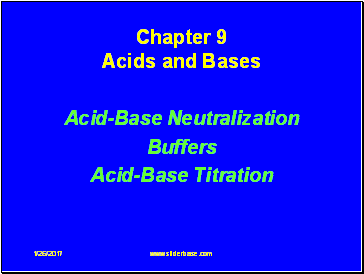1/26/2017

Chapter 9 Acids and Bases

Acid-Base Neutralization

Buffers

Acid-Base Titration

Slide 2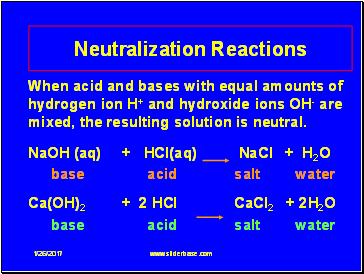## Neutralization Reactions

1/26/2017

When acid and bases with equal amounts of hydrogen ion H+ and hydroxide ions OH- are mixed, the resulting solution is neutral.

NaOH (aq) + HCl(aq) NaCl + H2O

base acid salt water

Ca(OH)2 + 2 HCl CaCl2 + 2H2O

base acid salt water

Slide 3## Neutralization

1/26/2017

H3O+ and OH- combine to produce water

H3O+ + OH-  2 H2O

from acid from base neutral

Net ionic equation:

H+ + OH-  H2O

Slide 4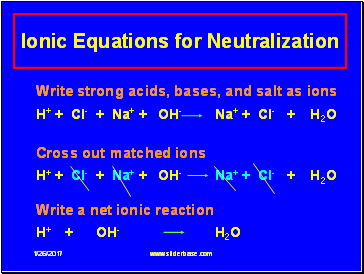## Ionic Equations for Neutralization

1/26/2017

Write strong acids, bases, and salt as ions

H+ + Cl- + Na+ + OH- Na+ + Cl- + H2O

Cross out matched ions

H+ + Cl- + Na+ + OH- Na+ + Cl- + H2O

Write a net ionic reaction

H+ + OH- H2O

Slide 5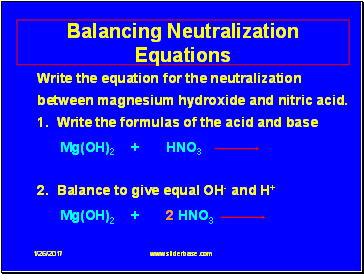## Balancing Neutralization Equations

1/26/2017

Write the equation for the neutralization between magnesium hydroxide and nitric acid. 1. Write the formulas of the acid and base

Mg(OH)2 + HNO3

2. Balance to give equal OH- and H+

Mg(OH)2 + 2 HNO3

Slide 6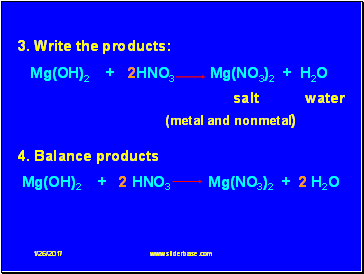1/26/2017

3. Write the products:

Mg(OH)2 + 2HNO3 Mg(NO3)2 + H2O

salt water

(metal and nonmetal)

4. Balance products

Mg(OH)2 + 2 HNO3 Mg(NO3)2 + 2 H2O

Slide 7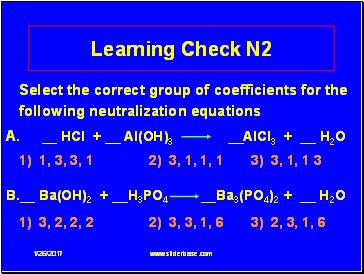Learning Check N2

1/26/2017

Select the correct group of coefficients for the following neutralization equations

A. HCl + Al(OH)3 AlCl3 + H2O

1) 1, 3, 3, 1 2) 3, 1, 1, 1 3) 3, 1, 1 3

B. Ba(OH)2 + H3PO4 Ba3(PO4)2 + H2O

1) 3, 2, 2, 2 2) 3, 3, 1, 6 3) 2, 3, 1, 6

Slide 8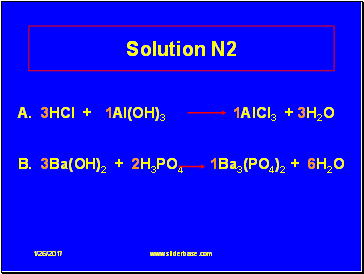1/26/2017

Solution N2

A. 3HCl + 1Al(OH)3 1AlCl3 + 3H2O

B. 3Ba(OH)2 + 2H3PO4 1Ba3(PO4)2 + 6H2O

Slide 91/26/2017

Learning Check N3

Write a balanced equation and calculate the mL of 2.00 M H2SO4 required to neutralize 50.0 mL of 1.00 M KOH?

_H2SO4 + _KOH _K2SO4 + H2O

1) 12.5 mL 2) 50.0 mL 3) 200. mL

Slide 10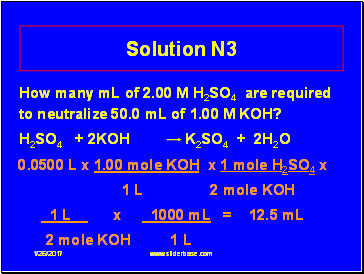1/26/2017

Go to page:
1  2